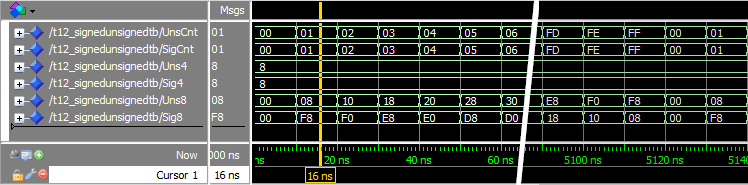# How to use Signed and Unsigned in VHDL

The signed and unsigned types in VHDL are bit vectors, just like the std_logic_vector type. The difference is that while the std_logic_vector is great for implementing data buses, it’s useless for performing arithmetic operations.

If you try to add any number to a std_logic_vector type, ModelSim will produce the compilation error: No feasible entries for infix operator +”. This is because the compiler doesn’t know how to interpret this collection of bits that the vector is.

This blog post is part of the Basic VHDL Tutorials series.

We must declare our vector as signed or unsigned for the compiler to treat it as a number.

The the syntax for declaring signed and unsigned signals is:
signal <name> : signed(<N-bits> downto 0) := <initial_value>;
signal <name> : unsigned(<N-bits> downto 0) := <initial_value>;

Just like with std_logic_vector, the ranges can be to or downto any range. But declaring signals with other ranges than downto 0 is so uncommon, that spending any more time on the subject would only serve to confuse us. The initial value is optional, by default it’s 'U' for all bits.

We have already been using the integer type for arithmetic operations in previous tutorials. So why do we need the signed and unsigned types? For most, digital designers like to have more control of how many bits a signal actually uses.

Also, signed and unsigned values wrap around, while the simulator will throw a run-time error if an integer is incremented beyond bounds. Finally, signed and unsigned can have other values like 'U' and 'X', while integers can only have number values. These meta-values can help us discovering errors in our design.

library ieee;
use ieee.std_logic_1164.all;
use ieee.numeric_std.all;

entity T12_SignedUnsignedTb is
end entity;

architecture sim of T12_SignedUnsignedTb is

signal UnsCnt : unsigned(7 downto 0) := (others => '0');
signal SigCnt : signed(7 downto 0) := (others => '0');

signal Uns4 : unsigned(3 downto 0) := "1000";
signal Sig4 : signed(3 downto 0) := "1000";

signal Uns8 : unsigned(7 downto 0) := (others => '0');
signal Sig8 : signed(7 downto 0) := (others => '0');

begin

process is
begin

wait for 10 ns;

-- Wrapping counter
UnsCnt <= UnsCnt + 1;
SigCnt <= SigCnt + 1;

Uns8 <= Uns8 + Uns4;
Sig8 <= Sig8 + Sig4;

end process;
end architecture;The radix of all signals in the waveform are set to hexadecimal so that we can compare them equally.

In the wrapping counter example, we see that the signed and unsigned signals behave exactly the same way. Both UnsCnt and SigCnt start at 0, and are incremented one-by-one up to FF. Hex FF (decimal 255) is the largest value our 8-bit signals can hold. Therefore, the next increment wraps both of them back to 0.

We created the two 4-bit signals Uns4 and Sig4, and gave them both an initial value of 1000”. We can see from the waveform that they are both just hex 8 (binary 1000).

The last two 8-bit signals we created were Uns8 and Sig8. We can see from the waveform that their initial values are 0, as one would expect. But from there, they behave differently! Apparently, signed and unsigned types made a difference when adding two signals of different lengths.

This is because of something known as sign extension. Adding positive or negative numbers stored in vectors of equal length, is the same operation in digital logic. This is because of how two’s complement works. If the vectors are of different lengths, the shortest vector will have to be extended.

The unsigned 4-bit binary number 1000” is decimal 8, while the signed 4-bit number 1000” is decimal -8. The 1” at the left-most place of the signed number indicates that this is a negative number. Therefore, the two 4-bit signals are sign extended differently by the compiler.

This is a visualization of how sign extension creates the differing values for the Uns8 and Sig8 signals:• Signals of signed and unsigned type are vectors that can be used in arithmetic operations
• Signals of signed and unsigned type will overflow silently
• Sign extension may create differing results for signed and unsigned types

## تبلیغات